Pythagorean Theorem
Special Right Triangles
Similar Right Triangles
Sine, Cosine, and Tangent
Solving Right Triangles
100

Solve for x. Round your answer to the nearest hundredth if necessary.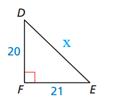29

100

Solve for x in the triangle shown. Write answers in exact form.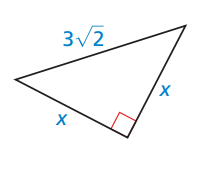x = 3

100

Name 2 triangles similar to triangle SRT.triangleSTU and triangleTRU

100

Name the sin, cos, and tan of angle S.sinS=28/53

cosS=45/53

tanS=28/45

100

What does it mean to solve a right triangle?

To find all angles and sides.

200

Solve for x. Round your answer to the nearest hundredth if necessary.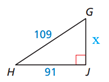60

200

Solve for x and y in the triangle shown. Write answers in exact form.x=16sqrt3, y=8sqrt3

200

Solve for aa=3

200

Find sin x and tan x, given that

cos x =5/7

sin x = sqrt(24)/7 or (2sqrt6)/7

tan x = sqrt(24)/5 or (2sqrt6)/5

200

Solve the triangle. Round to the nearest hundredth.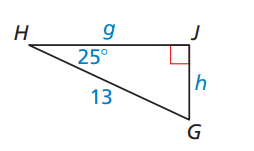mangleG=65

h=5.49

g=11.78

300

Determine whether the side lengths form a triangle. If so, is the triangle acute, obtuse, or right?

7, 11, 18

Not a triangle

300

The perimeter of a square is 68 meter. Find the length of the diagonal. Write exact answer.

17sqrt2

300

Solve for y. Round answer to the nearest tenth.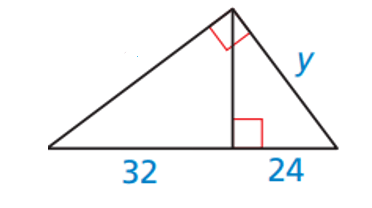y=36.7

300

From a hot-air balloon, Sasha measures a 38 degree angle of depression to a landmark that’s 1922 feet away, measuring horizontally. What’s the balloon’s vertical distance above the ground? Round your answer to the nearest hundredth of a foot if necessary.

1501.63 feet

300

Solve the triangle. Round answers to the nearest hundredth.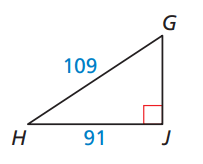GJ=60

mangleH=33.40

mangleG=56.60

400

Determine whether the side lengths form a triangle. If so, is the triangle acute, obtuse, or right?

3sqrt2,5,9

Obtuse

400

The perimeter of an equilateral triangle is 30 cm. What is the length of its altitude. Write the exact answer.

5sqrt3

400

Solve for x and y. Round answers to the nearest tenth if necessary.x=9 and y=15

400

If a person was standing 35 feet from a tree that is 43 feet tall, what is the measure of the angle of elevation to the nearest tenth?

50.9 degrees

400

Solve the triangle. Round to the nearest tenth.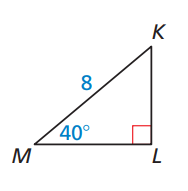mangleK=50

KL=5.1

ML=6.1

500

Determine the area of the triangle. Round to the nearest tenth.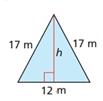95.4

500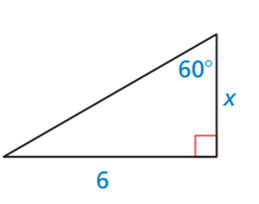x=2sqrt3

500

Solve for x. Round answer to the nearest tenth.x=18.5

500

If a person is flying a kite and they let the string out 95 feet with an angle of elevation at 44 degrees, what is their horizontal distance from the kite?

68.3 feet

500

Solve the triangle.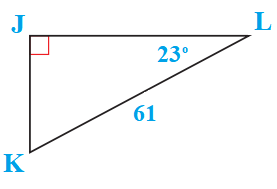mltK=67

JL=56.2

JK=23.8

Click to zoom